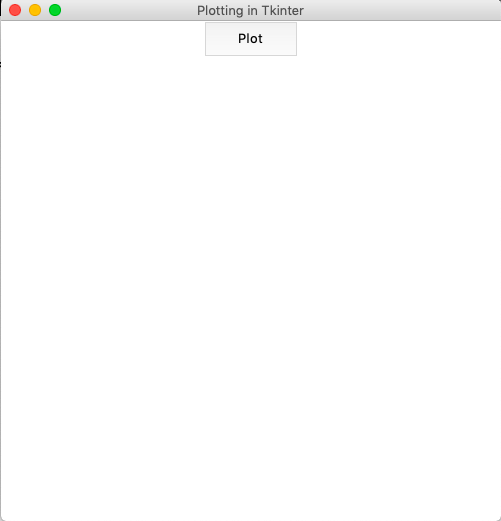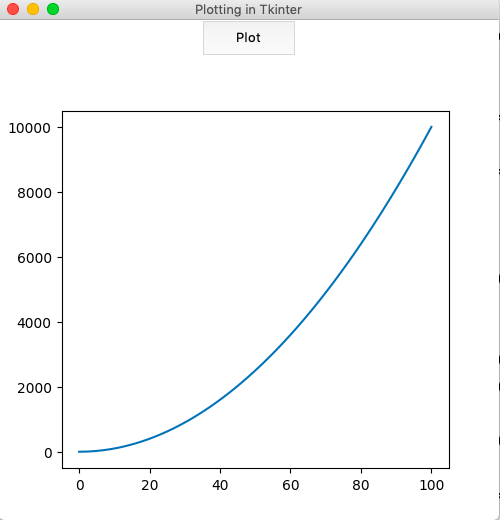Open in App
Not now

# How to embed Matplotlib charts in Tkinter GUI?

• Difficulty Level : Medium
• Last Updated : 10 Jul, 2020

Prerequisite: Introduction to Tkinter | Introduction to Matplotlib

When Matplotlib is used from Python shell, the plots are displayed in a default window. The plots can be embedded in many graphical user interfaces like wxpython, pygtk, or Tkinter. These various options available as a target for the output plot are referred to as ‘backends‘. There are various modules available in matplotlib.backend for choosing the backend. One such module is backend_tkagg which is useful for embedding plots in Tkinter.

#### Creating the Tkinter Application :

First, let us create a basic Tkinter application with the main window and one button which can be used to display the plot.

## Python3

 `# import all classes/methods``# from the tkinter module``from` `tkinter ``import` `*`` ` `# The main tkinter window``window ``=` `Tk()`` ` `# setting the title and ``window.title(``'Plotting in Tkinter'``)`` ` `# setting the dimensions of ``# the main window``window.geometry(``"500x500"``)`` ` `# button that would displays the plot``plot_button ``=` `Button(master ``=` `window,``                     ``height ``=` `2``,``                     ``width ``=` `10``,``                    ``text ``=` `"Plot"``)``# place the button``# into the window``plot_button.pack()`` ` `# run the gui``window.mainloop()`

Output :#### Embedding the Plot:

First, we need to create the figure object using the Figure() class. Then, a Tkinter canvas(containing the figure) is created using FigureCanvasTkAgg() class. Matplotlib charts by default have a toolbar at the bottom. When working with Tkinter, however, this toolbar needs to be embedded in the canvas separately using the NavigationToolbar2Tk() class.
In the implementation below, a simple graph for:is plotted. The plot function is bound to a button that displays the figure when pressed.

## Python3

 `from` `tkinter ``import` `*` `from` `matplotlib.figure ``import` `Figure``from` `matplotlib.backends.backend_tkagg ``import` `(FigureCanvasTkAgg, ``NavigationToolbar2Tk)`` ` `# plot function is created for ``# plotting the graph in ``# tkinter window``def` `plot():`` ` `    ``# the figure that will contain the plot``    ``fig ``=` `Figure(figsize ``=` `(``5``, ``5``),``                 ``dpi ``=` `100``)`` ` `    ``# list of squares``    ``y ``=` `[i``*``*``2` `for` `i ``in` `range``(``101``)]`` ` `    ``# adding the subplot``    ``plot1 ``=` `fig.add_subplot(``111``)`` ` `    ``# plotting the graph``    ``plot1.plot(y)`` ` `    ``# creating the Tkinter canvas``    ``# containing the Matplotlib figure``    ``canvas ``=` `FigureCanvasTkAgg(fig,``                               ``master ``=` `window)  ``    ``canvas.draw()`` ` `    ``# placing the canvas on the Tkinter window``    ``canvas.get_tk_widget().pack()`` ` `    ``# creating the Matplotlib toolbar``    ``toolbar ``=` `NavigationToolbar2Tk(canvas,``                                   ``window)``    ``toolbar.update()`` ` `    ``# placing the toolbar on the Tkinter window``    ``canvas.get_tk_widget().pack()`` ` `# the main Tkinter window``window ``=` `Tk()`` ` `# setting the title ``window.title(``'Plotting in Tkinter'``)`` ` `# dimensions of the main window``window.geometry(``"500x500"``)`` ` `# button that displays the plot``plot_button ``=` `Button(master ``=` `window, ``                     ``command ``=` `plot,``                     ``height ``=` `2``, ``                     ``width ``=` `10``,``                     ``text ``=` `"Plot"``)`` ` `# place the button ``# in main window``plot_button.pack()`` ` `# run the gui``window.mainloop()`

Output :My Personal Notes arrow_drop_up